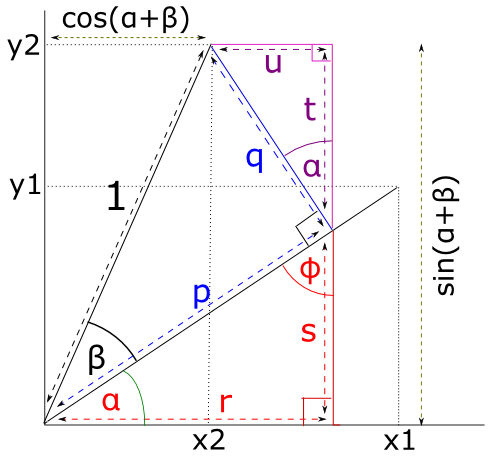$$\newcommand{L}{\| #1 \|}\newcommand{VL}{\L{ \vec{#1} }}\newcommand{R}{\operatorname{Re}\,(#1)}\newcommand{I}{\operatorname{Im}\, (#1)}$$

# The angle sum rule¶

The angle sum rule is:

\begin{align}\begin{aligned}\sin(\alpha \pm \beta) = \sin \alpha \cos \beta \pm \cos \alpha \sin \beta\\\cos(\alpha \pm \beta) = \cos \alpha \cos \beta \mp \sin \alpha \sin \beta\end{aligned}\end{align}

## Proof¶

Let’s say we have a vector $$(x_1, y_1)$$ resulting from the anticlockwise rotation of a length 1 vector $$(1, 0)$$ by $$\alpha$$ degrees around the origin.

We rotate this vector another $$\beta$$ degrees anticlockwise around the origin to give length 1 vector $$(x_2, y_2)$$.We can see from the picture that:

\begin{align}\begin{aligned}\cos(\alpha + \beta) = x_2 = r - u\\\sin(\alpha + \beta) = y_2 = t + s\end{aligned}\end{align}

We are going to use some basic trigonometry to get the lengths of $$r, u, t, s$$.

Because the angles in a triangle sum to 180 degrees, $$\phi$$ on the picture is $$90 - \alpha$$ and therefore the angle between lines $$q, t$$ is also $$\alpha$$.

Remembering the definitions of $$\cos$$ and $$\sin$$:

\begin{align}\begin{aligned}\cos\theta = \frac{A}{H} \implies A = (\cos \theta) H\\\sin\theta = \frac{O}{H} \implies O = (\sin \theta) H\end{aligned}\end{align}

Thus:

\begin{align}\begin{aligned}p = \cos \beta\\q = \sin \beta\\r = (\cos \alpha) p = \cos \alpha \cos \beta\\s = (\sin \alpha) q = \sin \alpha \cos \beta\\t = (\cos \alpha) q = \cos \alpha \sin \beta\\u = (\sin \alpha) q = \sin \alpha \sin \beta\end{aligned}\end{align}

So:

\begin{align}\begin{aligned}\cos(\alpha + \beta) = x_2 = r - u = \cos \alpha \cos \beta - \sin \alpha \sin \beta\\\sin(\alpha + \beta) = y_2 = t + s = \sin \alpha \cos \beta + \cos \alpha \sin \beta\end{aligned}\end{align}